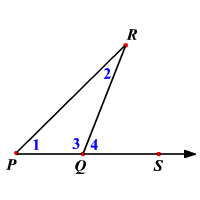# Exterior Angle Theorem

The measure of an exterior angle of a triangle is equal to the sum of the measures of the two non-adjacent interior angles of the triangle.$m\angle 4=m\angle 1+m\angle 2$

Proof:

Given: $\Delta PQR$

To Prove: $m\angle 4=m\angle 1+m\angle 2$

 Statement Reason 1 $\Delta PQR$ is a triangle Given 2 $m\angle 1+m\angle 2+m\angle 3=180°$ Triangle Sum Theorem 3 $\angle 3$ and $\angle 4$ form a linear pair Definition of linear pair. 4 $\angle 3$ and $\angle 4$ are supplementary If two angles form a linear pair, they are supplementary. 5 $m\angle 3+m\angle 4=180°$ Definition of supplementary angles. 6 $m\angle 3+m\angle 4=m\angle 1+m\angle 2+m\angle 3$ Statements 2, 5 and Substitution Property. 7 $m\angle 4=m\angle 1+m\angle 2$ Subtraction Property.